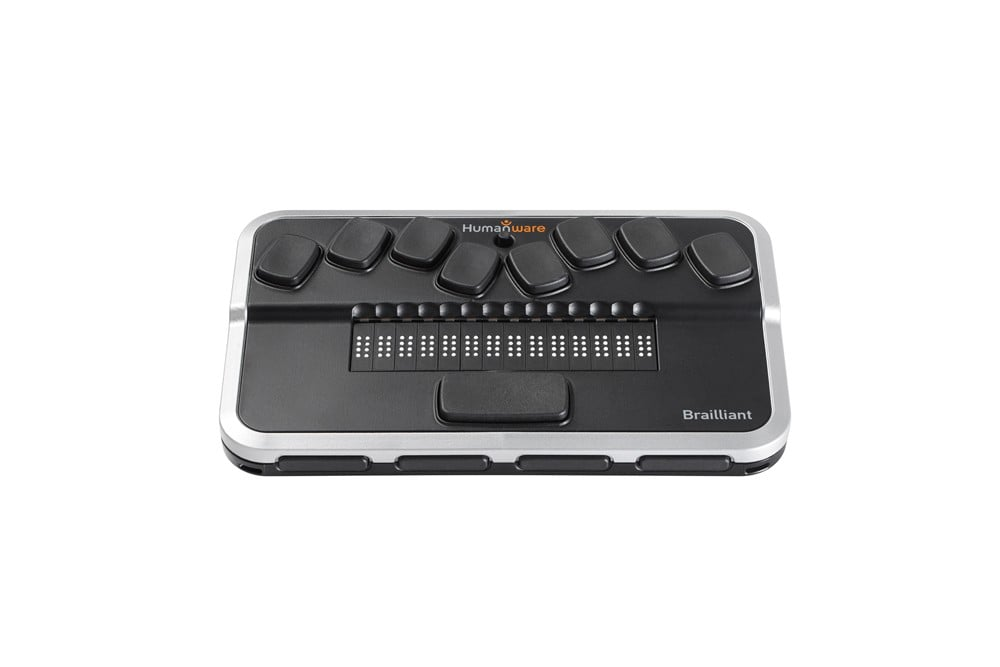The default button assignments for the HumanWare Brailliant BI 14 Braille display are listed below.

You can also discover what your Braille display buttons do by using SuperNova's and ScreenReader's Key Describe Modes.

To use Key Describe Mode, simply press CAPS LOCK + FORWARD SLASH and then press the buttons or button combinations on the display to find out what they do.

To leave Key Describe Mode, press CAPS LOCK + FORWARD SLASH again.

## Braille:

Line up = Up
Line down = Down
Line Start = Up + Left
Line End = Right + Down
Tracking on off = Left + Right
Physical/Logical Mode = Dot 2 + Dot 5 + Space
Literary Braille on off = Dot 1 + Dot 2 + Dot 4 + Dot 5 + Space

## Speak Keys:

Sentence Move to Next = Dot 1 + Dot 3 + Dot 5 + Space
Sentence Move to Previous = Dot 2 + Dot 4 + Dot 6 + Space

## Simulate Key Press:

Up = Dot 1 + Space
Down = Dot 4 + Space
Left = Dot 3 + Space
Right = Dot 6 + Space
Home = Dot 2 + Dot 3 + Space
End = Dot 5 + Dot 6 + Space
Ctrl Home = Dot 1 + Dot 2 + Dot 3 + Space
Ctrl End = Dot 4 + Dot 5 + Dot 6 + Space
Enter = Dot 8
Space = Space
Page Up = Dot 1 + Dot 2 + Space
Page Down = Dot 4 + Dot 5 + Space
Tab = Dot 4 + Dot 6 + Space
Shift Tab = Dot 1 + Dot 3 + Space
Ctrl Tab = Dot 1 + Dot 3 + Dot 4 + Dot 5 + Space
Ctrl Shift Tab = Dot 1 + Dot 2 + Dot 3 + Dot 4 + Space
Escape = Dot 1 + Dot 5 + Space
Backspace = Dot 7
Delete = Dot 1 + Dot 4 + Dot 5 + Space
Windows = Dot 3 + Dot 4 + Space
Alt = Dot 1 + Dot 3 + Dot 4 + Space

Up = Joystick Up
Down = Joystick Down
Left = Joystick Left
Right = Joystick Right
Enter = Joystick Action